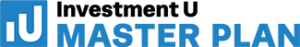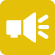## 1-Minute Survey

What Type of Investor Are You? – Take This 1-Min Survey to Find Out

Financial Literacy

# 4 Depreciation Expense Methods with Formulas and Examples

Depreciation expense can be a big portion of a company’s total expense. And since expenses decrease income, it affects the overall value of a company. Understanding what it is and the methods can help you determine if a company is as good in real life as it is on paper.  That’s why I’ve put together this guide with depreciation expense methods, formulas and examples.

## What is Depreciation Expense?

Depreciation is the decrease of a tangible asset’s value. It occurs over the span of its estimated useful life. This lets a company gradually write off the asset. To record depreciation, a company will debit an account called depreciation expense. This decreases the company’s income.

But depreciation doesn’t only affect companies. It affects everyone, including you. For example, you might have heard the advice to never buy a brand-new car. That’s because the second you drive off the lot, its value can drop 10% or more. And that doesn’t include the additional 10-20% you lose in the first year. Though, unlike companies, you don’t have to record depreciation expense.

There are a few different methods to calculate depreciation expense. Let’s take a look…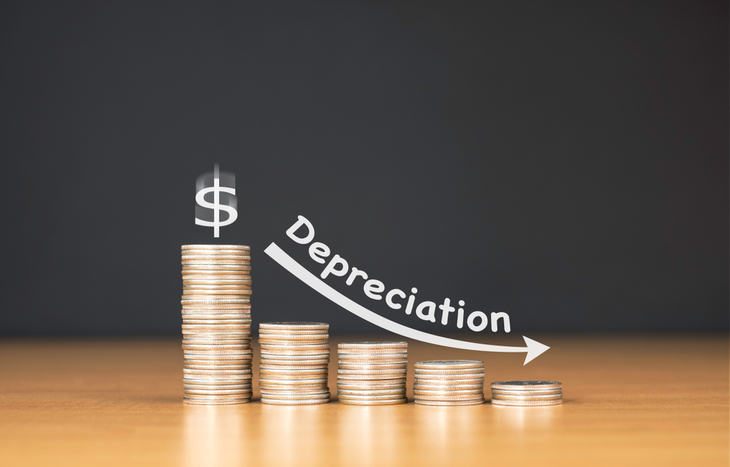## What are the Depreciation Methods?

There are four common methods to calculate depreciation:

• Straight-line
• Double-declining balance
• Sum-of-years digits
• Units of production

Each method is selected to fit the asset’s nature. Below you can find a description of each method and the types of assets it’s typically used for.

## Straight-Line

Straight-line depreciation is common and the easiest to calculate. In the method, the asset is depreciated by the same amount every year of its useful life.

Depreciation Expense = (Fair Value – Salvage Value) / Useful Life

The fair value is what you bought the asset for. The salvage value is what the asset is worth at the end of its useful life. This is also known as residual value. Let’s look at an example…

You buy a building for \$30 million. You expect the building to last 12 years with a salvage value of \$6 million. Subtract the \$6 million from \$30 million. The answer is \$24 million. That means that over the next 12 years, the building will depreciate by \$24 million. But rather than happening all at once, the depreciation is spread out across the 12 years. So, take \$24 million and divide it by 12.

The annual depreciation expense is \$2 million.

(30 million – 6 million) / 12 = 2

The straight-line method can be applied to most assets. If there isn’t a specific trend to the asset’s use, straight-line depreciation is applied.

## Double-Declining Balance

This method is used when the asset depreciates more quickly in the earlier years, like your car. It has a 2x factor to accelerate depreciation. In straight-line, the factor is 1. But that doesn’t mean you multiply the straight-line by two. The formula is as followed:

Depreciation Expense = (Beginning Book Value for Year * 2) / Useful Life

Let’s use a car for an example. You buy a car for \$50,000. It has a useful life of 5 years. In the first year, the depreciation would be calculated like this:

(50,000 * 2) / 5 = 20,000

For the first year, the depreciation expense is \$20,000. This decreases the book value to \$30,000. When calculating the second year’s depreciation, \$30,000 is the value you use.

Year 2- (30,000 * 2) / 5 = 12,000

Year 3- (18,000 * 2) / 5 = 7,200

Year 4- (10,800 * 2) / 5 = 4,320

Year 5- (5,680 * 2) / 5 = 2,272

Net Book Value at end of Useful Life = \$3,408

However, note that assets typically have a salvage value. If your car’s salvage value is \$3,500, then don’t depreciate the full \$2,272 in the fifth year. It depreciates your car past its value. Instead, depreciate \$2,180 to bring the value to \$3,500.

## Sum-of-Years Digits

Sum-of-years digits (SYD) applies a percentage to determine the depreciation value. Like double-declining, this is an accelerated method. To calculate SYD, first determine the asset’s useful life. Then, find the sum of the digits leading to that number. This will be your base.

For example, you purchase a machine for \$100,000. Its salvage value is \$10,000. Its useful life is five years. To find the base, add all the numbers from one to five.

1 + 2 + 3 + 4 + 5 = 15

Now, take the number of years left in the useful life and divide it by the base. So, if it’s the first year, there are five years left the machine is usable. This will give you a percentage.

5 / 15 = 33.33%

You then take that percentage and apply it to the depreciation value. In this case, that’s \$90,000 because it’s worth \$10,000 after the five years. So, 33.33% of \$90,000 is \$30,000.

For the second year, there are four years remaining in the useful life.

4 / 15 = 26.67%

The depreciation value doesn’t change. That means you still take a percentage of \$90,000. And 29.67% of \$90,000 is about \$24,000.

This will continue until the end of the asset’s useful life. By then, the percentages should total 100% for a total depreciation of \$90,000.

## Units of Production

Units of production is a method of depreciation based on asset usage. It’s mostly used by manufacturers because their machinery’s usage varies with customer demands. Depreciating by units produced better matches expenses and revenues. It also is a more accurate representation of wear on the machinery or equipment.

Let’s take the example above. You buy a machine for \$100,000 with a salvage value of \$10,000. While you should know the useful life, it’s not needed to determine the depreciation expense. Instead, you estimate how many units the machine will produce over the span of its useful life. Let’s say it will produce 45,000 units over five years.

Then, you determine the depreciation cost per unit.

(100,000 – 10,000) / 45,000 = 2

For every unit produced, it will cost \$2 of depreciation. To determine the depreciation cost for one year, you multiply \$2 by the number of units produced in the given year. If the machine produces 10,000 units in the first year, the depreciation expense is \$20,000. If the machine produces 15,000 units in the second year, the depreciation expense is \$30,000. This method can also be used with hours of usage instead of units produced. In the above example, it would be 45,000 hours over its useful life.

With a great knowledge of depreciation expense, you’re better prepared for investment decisions. Check out our Investment Opportunities page to get started. And don’t forget to sign up for our free e-letter below! It has great investment tips and advice.

Amber Deter has researched and written about initial public offerings (IPOs) over the last few years. After starting her college career studying accounting and business, Amber decided to focus on her love of writing. Now she’s able to bring that experience to Investment U readers by providing in-depth research on IPO and investing opportunities.

###### Articles by Amber DeterInvestment Opportunities

June 10, 2021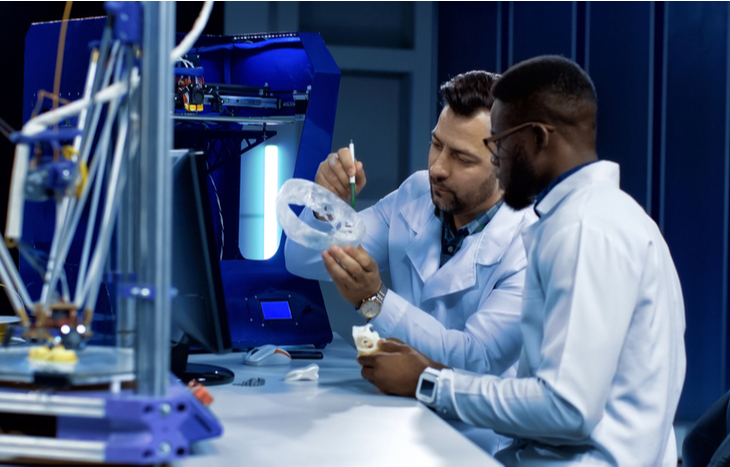Investment Opportunities
###### Relativity Space Stock: Will This SpaceX Competitor Go Public?

June 9, 2021Investment Opportunities
###### Krispy Kreme Stock Is Almost Here: What to Know About the DNUT IPO

June 2, 2021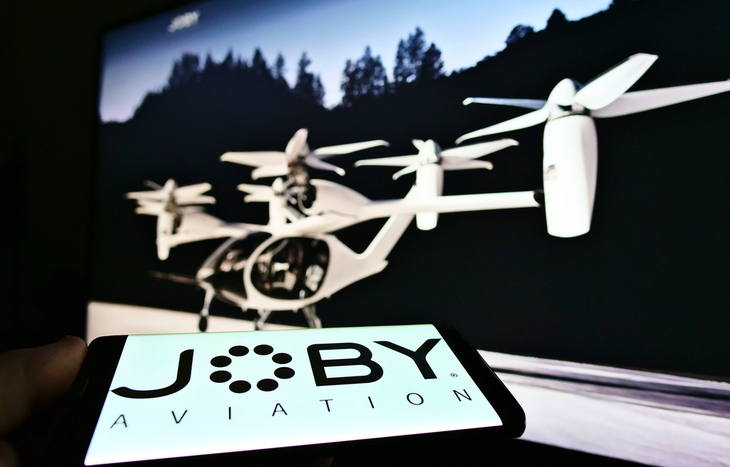Investment Opportunities
###### Joby Aviation Stock Is Almost Here: What to Know About the SPAC IPO

May 28, 2021